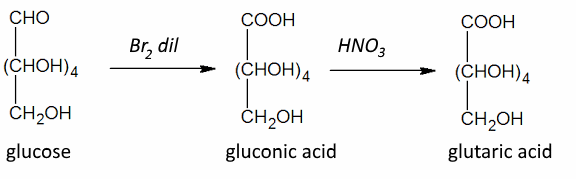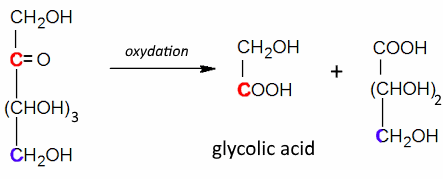﻿Search:

# Oxidation of hexoses

## Aldohexoses

Often: Weak oxidizing agents: Position 1 : $- CHO$ ​​→ $-COOH$ Strong oxidizing agents: Position 1 : $-CHO$ ​​→ $-COOH$ and position 6 : $-CH_2OH$ → $-COOH$ ($\omega$-oxidation) Strong oxidizing agents: and protected position: 1 Position 6 : $-CH_2OH$ → $-COOH$ ($\omega$-oxidation)

ExamplesGluconic acid is naturally present in many fruits. It has many uses in dietetics, medicine and industry!Such protection is often in the form of acetals $CHO$ ​​→ $R^1-O-CH-O-R^2$

## Ketohexoses

Often: Low Oxidizers: Rupture between positions 2 and 3 and oxidation of these positions to $-COOH$